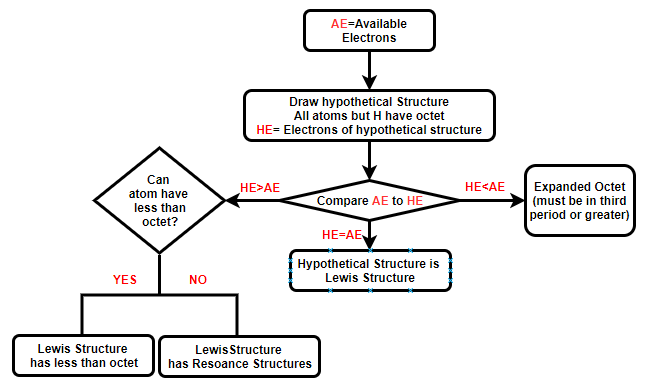# 8.5: Drawing Lewis Dot Structures

$$\newcommand{\vecs}{\overset { \rightharpoonup} {\mathbf{#1}} }$$ $$\newcommand{\vecd}{\overset{-\!-\!\rightharpoonup}{\vphantom{a}\smash {#1}}}$$$$\newcommand{\id}{\mathrm{id}}$$ $$\newcommand{\Span}{\mathrm{span}}$$ $$\newcommand{\kernel}{\mathrm{null}\,}$$ $$\newcommand{\range}{\mathrm{range}\,}$$ $$\newcommand{\RealPart}{\mathrm{Re}}$$ $$\newcommand{\ImaginaryPart}{\mathrm{Im}}$$ $$\newcommand{\Argument}{\mathrm{Arg}}$$ $$\newcommand{\norm}{\| #1 \|}$$ $$\newcommand{\inner}{\langle #1, #2 \rangle}$$ $$\newcommand{\Span}{\mathrm{span}}$$ $$\newcommand{\id}{\mathrm{id}}$$ $$\newcommand{\Span}{\mathrm{span}}$$ $$\newcommand{\kernel}{\mathrm{null}\,}$$ $$\newcommand{\range}{\mathrm{range}\,}$$ $$\newcommand{\RealPart}{\mathrm{Re}}$$ $$\newcommand{\ImaginaryPart}{\mathrm{Im}}$$ $$\newcommand{\Argument}{\mathrm{Arg}}$$ $$\newcommand{\norm}{\| #1 \|}$$ $$\newcommand{\inner}{\langle #1, #2 \rangle}$$ $$\newcommand{\Span}{\mathrm{span}}$$

## Introduction

There are multiple ways to draw Lewis dot structures. We will follow a flow chart that allows you to identify exceptions to octet rules and resonance structures. You start by counting the number of electrons the atoms donate to the structure, and you need to take into account charge if you are drawing an ion. Then draw a hypothetical structure where every atom except hydrogen has an octet, and then count the number of electrons in the structure. It is either less than, equal or greater than the number of electrons donated. These are outlined in the following flow chart and step-by-step rules are given below.Figure $$\PageIndex{1}$$: Flow chart for logic involved in the Lewis dot structure technique (below)

## Lewis Dot Structure Technique

The following handout has these instructions: http://chemwiki.ucdavis.edu/@api/deki/files/60817/vsepr-1-Review.pdf

1. Determine total number of available valence electrons.

• Determine the total number of valence electrons available, each atoms of the representative elements contributes the number of electrons equal to it’s group number (IA – VIIA).
• If there is an odd number of electrons, the structure will be a free radical, which has a lone electron in an orbital. This electron will exist in a nonbonding orbital or a multiple bond.
• If the compound is an ion,
• add one electron for each negative charge
• subtract one electron for each positive charge

2. Determine structural connectivity.

• First atom is central atom (unless Hydrogen, which can never be central)
• If more than 2 types of atoms, set up in order of formula
• Organic compounds often contain multiple carbons connected to each other, you will need to be able to identify functional groups.

3. Draw hypothetical structure with each atom surrounded by 8 electrons in pairs.

• Place two electrons forming a single bond between bonded atoms by drawing a line between the two atoms.
• For each atom not surrounded by an octet of electrons (4 bonds), add lone pairs of electrons (two dots to a lone pair) until all atoms are surrounded by an octet.
• Note, hydrogen can only have 2 valence electrons.

4. Count the total number of electrons in the hypothetical structure.

1. If it is EQUAL to the number of available electrons from step 1, this is the correct Lewis Dot Structure.
2. If it is GREATER than the number of available electrons from step 1, go to step 5.
3. If it is LESS than the available number of electrons from step 1, go to step 7.

5. Determine if any atoms can have less than an octet.

If not, proceed to step 6, if so, decrease the number of loan pairs around these atoms until the total number of electrons in structure equals the number from step 1.

a. Hydrogen always has a duet (one bond – 2 electrons)

b. Group IIA can have a quartet (4 electrons)

c. Group IIIA can have a sextet (6 electrons)

6. Combine adjacent lone pairs into multiple bonds

Place multiple bonds into structure by replacing two loan pairs from two adjacent atoms with a bonding pair. Resonance structures result if you have more than one option for doing this. Repeat this until the structure has the same number of electrons as determined in step 1. Be sure to generate all resonance structures

7. Expanded Octets

If any atoms have d orbitals available (3rd period or greater), they can have an “expanded octet”, that is more than 8 valence electrons. Add lone pairs to these atoms until the number of electrons equals the number from step 1.

8 RESONANCE STRUCTURES: Check step 6 to see if additional electron configurations can be drawn by changing the position of multiple bonds, these represent resonance structures. The best configurations will be typically be the ones where the atoms are assigned the formal charges closest to zero (step 9). In the event that no resonance structure has a zero formal charge, assign negative formal charges to the most electronegative elements.

9. FORMAL CHARGES: These correlate the electron locations in the molecule to the atom that donates them. The formal charge (FC) for each atom can be determined by the following equation. The sum of the formal charges equals the charge of the species.

## Examples

### Sulfite

Worksheet

The following worksheet should be downloaded and filled out. This is the first of two companion worksheets and in the molecular geometry we will start with the Lewis dot structures of these molecules and determine their geometry's, polarity's and the types of molecular orbitals involved in the bonds.

Handout 1.2: Lewis Dot Structure Worksheet:
http://chemwiki.ucdavis.edu/@api/deki/files/61154/vsepr1_WorkSheet.pdf

8.5: Drawing Lewis Dot Structures is shared under a not declared license and was authored, remixed, and/or curated by Robert Belford.